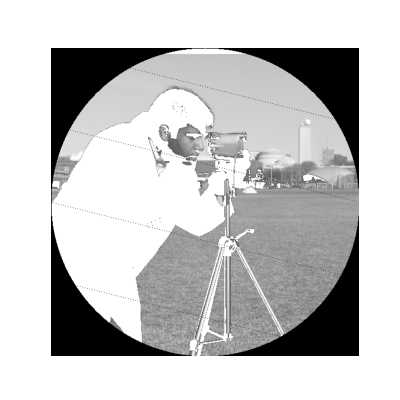# Using simple NumPy operations for manipulating images#

This script illustrates how to use basic NumPy operations, such as slicing, masking and fancy indexing, in order to modify the pixel values of an image.```import numpy as np
from skimage import data
import matplotlib.pyplot as plt

camera = data.camera()
camera[:10] = 0
inds_x = np.arange(len(camera))
inds_y = (4 * inds_x) % len(camera)
camera[inds_x, inds_y] = 0

l_x, l_y = camera.shape, camera.shape
X, Y = np.ogrid[:l_x, :l_y]
outer_disk_mask = (X - l_x / 2)**2 + (Y - l_y / 2)**2 > (l_x / 2)**2